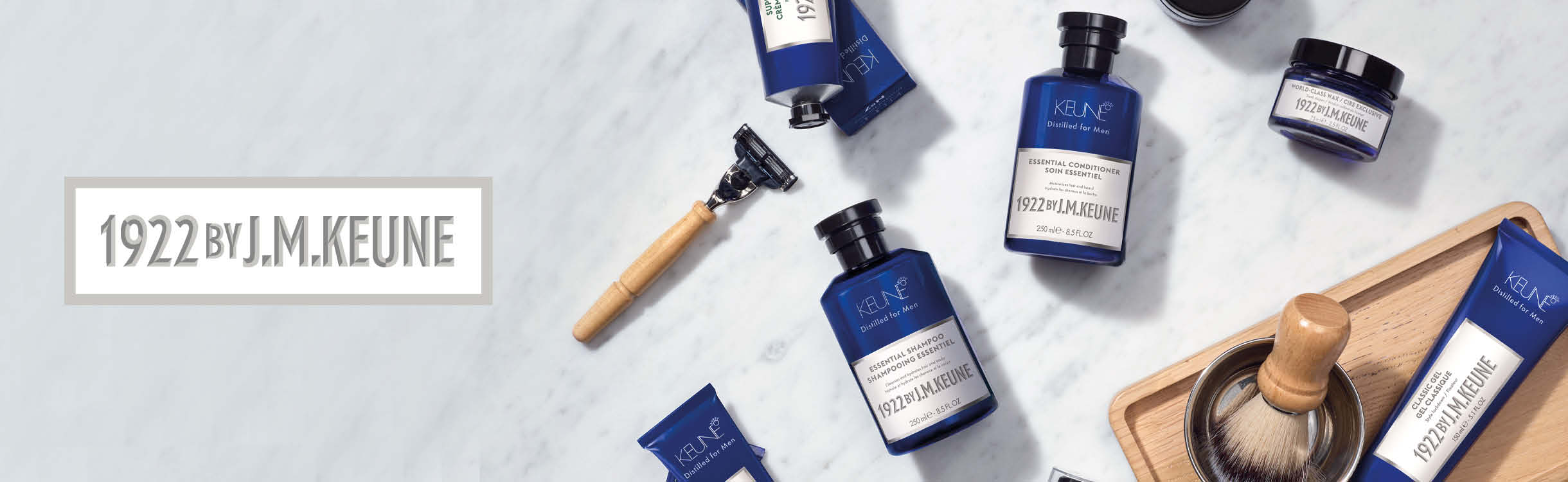# 1922 by J. M. Keune

1922 by J. M. Keune traz produtos que tornam a rotina dos homens mais prática. A linha conta com todos os itens para cuidar da barba, cabelo e bigode.

## Todos os Produtos

-13%STOP na quedaR\$ 153,90
R\$ 132,90
4x de R\$ 33,23
-37%STOP na quedaR\$ 413,90
R\$ 256,90
8x de R\$ 32,11
Frete Grátis
-37%R\$ 159,90
R\$ 99,90
3x de R\$ 33,30
-38%STOP na quedaR\$ 83,90
R\$ 51,90
-14%R\$ 83,90
R\$ 71,90
2x de R\$ 35,95
-37%R\$ 413,90
R\$ 256,90
8x de R\$ 32,11
Frete Grátis
-39%R\$ 182,90
R\$ 110,90
3x de R\$ 36,97
-39%R\$ 196,90
R\$ 119,90
3x de R\$ 39,97
-35%R\$ 336,80
R\$ 215,80
7x de R\$ 30,83
Frete Grátis
-39%R\$ 182,90
R\$ 110,90
3x de R\$ 36,97
-31%R\$ 153,90
R\$ 104,90
3x de R\$ 34,97
-13%R\$ 153,90
R\$ 132,90
4x de R\$ 33,23
-48%OutletR\$ 196,90
R\$ 101,90
3x de R\$ 33,97
-38%R\$ 83,90
R\$ 51,90
-37%R\$ 413,90
R\$ 256,90
8x de R\$ 32,11
Frete Grátis
-39%R\$ 106,90
R\$ 64,90
2x de R\$ 32,45
-38%R\$ 87,90
R\$ 53,90
-39%VeganoR\$ 265,90
R\$ 160,90
5x de R\$ 32,18
-38%Cruelty FreeR\$ 83,90
R\$ 51,90
-43%OutletR\$ 350,80
R\$ 197,80
6x de R\$ 32,97
Frete Grátis
-39%R\$ 221,90
R\$ 133,90
4x de R\$ 33,48
-14%R\$ 103,90
R\$ 88,90
2x de R\$ 44,45
-13%R\$ 215,90
R\$ 186,90
6x de R\$ 31,15
-37%R\$ 153,90
R\$ 95,90
3x de R\$ 31,97
-13%R\$ 196,90
R\$ 169,90
5x de R\$ 33,98
-24%R\$ 350,80
R\$ 265,80
8x de R\$ 33,23
Frete Grátis
-13%R\$ 426,90
R\$ 369,90
10x de R\$ 36,99
Frete Grátis
-13%R\$ 382,90
R\$ 332,90
10x de R\$ 33,29
Frete Grátis
-13%R\$ 181,90
R\$ 157,90
5x de R\$ 31,58
-13%R\$ 196,90
R\$ 169,90
5x de R\$ 33,98
-13%R\$ 413,90
R\$ 358,90
10x de R\$ 35,89
Frete Grátis
-13%R\$ 330,90
R\$ 286,90
9x de R\$ 31,88
Frete Grátis
-25%STOP na quedaR\$ 796,80
R\$ 589,80
10x de R\$ 58,98
Frete Grátis
-13%R\$ 306,90
R\$ 265,90
8x de R\$ 33,24
Frete Grátis
-25%R\$ 796,80
R\$ 589,80
10x de R\$ 58,98
Frete Grátis
-13%R\$ 343,90
R\$ 298,90
9x de R\$ 33,21
Frete Grátis
Você está offline.